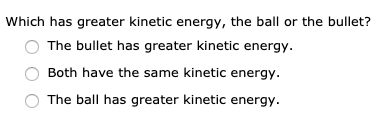# Which has greater kinetic energy, the ball or the bullet?The bullet has greater kinetic energy.Both have the same kinetic energy.The ball has greater kinetic energy.

Question
63 views

A bullet with a mass of 3.18 g moves at a speed of 1.50 ✕ 103 m/s. If a tennis ball of mass 58.4 g has the same momentum as the bullet, what is its speed (in m/s)?help_outlineImage TranscriptioncloseWhich has greater kinetic energy, the ball or the bullet? The bullet has greater kinetic energy. Both have the same kinetic energy. The ball has greater kinetic energy. fullscreen
check_circle

Step 1

Given information:

The mass of the bullet (mb) = 3.18 g = 0.0038 kg

The bass of the tennis ball (mt) = 58.4 g = 0.0584 kg

The velocity of the bullet (vb) = 1.5 ×103 m/s

Let the velocity of the tennis ball = vt

Step 2

Given the ball and the bullet have the same momentum hence we can write:

Step 3

The kinetic energy of the ...

### Want to see the full answer?

See Solution

#### Want to see this answer and more?

Solutions are written by subject experts who are available 24/7. Questions are typically answered within 1 hour.*

See Solution
*Response times may vary by subject and question.
Tagged in